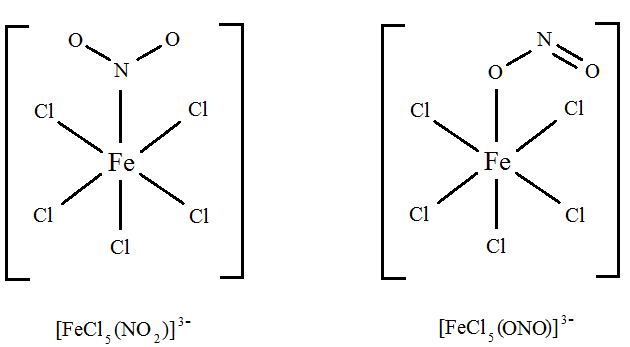# 5.9: Structural Isomers- Linkage Isomerism in Transition Metal Complexes

$$\newcommand{\vecs}{\overset { \rightharpoonup} {\mathbf{#1}} }$$ $$\newcommand{\vecd}{\overset{-\!-\!\rightharpoonup}{\vphantom{a}\smash {#1}}}$$$$\newcommand{\id}{\mathrm{id}}$$ $$\newcommand{\Span}{\mathrm{span}}$$ $$\newcommand{\kernel}{\mathrm{null}\,}$$ $$\newcommand{\range}{\mathrm{range}\,}$$ $$\newcommand{\RealPart}{\mathrm{Re}}$$ $$\newcommand{\ImaginaryPart}{\mathrm{Im}}$$ $$\newcommand{\Argument}{\mathrm{Arg}}$$ $$\newcommand{\norm}{\| #1 \|}$$ $$\newcommand{\inner}{\langle #1, #2 \rangle}$$ $$\newcommand{\Span}{\mathrm{span}}$$ $$\newcommand{\id}{\mathrm{id}}$$ $$\newcommand{\Span}{\mathrm{span}}$$ $$\newcommand{\kernel}{\mathrm{null}\,}$$ $$\newcommand{\range}{\mathrm{range}\,}$$ $$\newcommand{\RealPart}{\mathrm{Re}}$$ $$\newcommand{\ImaginaryPart}{\mathrm{Im}}$$ $$\newcommand{\Argument}{\mathrm{Arg}}$$ $$\newcommand{\norm}{\| #1 \|}$$ $$\newcommand{\inner}{\langle #1, #2 \rangle}$$ $$\newcommand{\Span}{\mathrm{span}}$$

Linkage isomerism occurs with ambidentate ligands that are capable of coordinating in more than one way. The best known cases involve the monodentate ligands: $$SCN^- / NCS^-$$ and $$NO_2^- / ONO^-$$. The only difference is what atoms the molecular ligands bind to the central ion. The ligand(s) must have more than one donor atom, but bind to ion in only one place. For example, the (NO2-) ion is a ligand can bind to the central atom through the nitrogen or the oxygen atom, but cannot bind to the central atom with both oxygen and nitrogen at once, in which case it would be called a polydentate rather than an ambidentate ligand.Figure $$\PageIndex{1}$$: Linkage Isomerism in the $$NO_2$$ ligand. This occurs when a particular ligand is capable of coordinating to a metal in two different and distinct ways. from Angel C. de Dios.

The names used to specify the changed ligands are changed as well. For example, the (NO2-) ion is called nitro when it binds with the N atom and is called nitrito when it binds with the O atom.

Example $$\PageIndex{1}$$: Nitro- vs. Nitrito- Linkage Isomers

The cationic cobalt complex [Co(NH3)5(NO2)]Cl2 exists in two separable linkage isomers of the complex ion: (NH3)5(NO2)]2+.(left) The nitro isomer (Co-NO2) and (right) the nitrito isomer (Co-ONO)

When donation is from nitrogen to a metal center, the complex is known as a nitro- complex and when donation is from one oxygen to a metal center, the complex is known as a nitrito- complex. An alternative formula structure to emphasize the different coordinate covalent bond for the two isomers

• $$[Co(ONO)(NH_3)_5]Cl$$: the nitrito isomer -O attached
• $$[Co(NO_2)(NH_3)_5]Cl$$: the nitro isomer - N attached.

The formula of the complex is unchanged, but the properties of the complex may differ.

Another example of an ambidentate ligans is thiocyanate, SCN, which can attach at either the sulfur atom or the nitrogen atom. Such compounds give rise to linkage isomerism. Polyfunctional ligands can bond to a metal center through different ligand atoms to form various isomers. Other ligands that give rise to linkage isomers include selenocyanate, SeCN – isoselenocyanate, NCSeand sulfite, SO32−.

Exercise $$\PageIndex{1}$$

Are [FeCl5(NO2)]3– and [FeCl5(ONO)]3– linkage isomers?

Solution

Here, the difference is in how the ligand bonds to the metal. In the first isomer, the ligand bonds to the metal through an electron pair on the nitrogen. In the second isomer, the ligand bonds to the metal through an electron pair on one of the oxygen atoms. It's easier to see it: